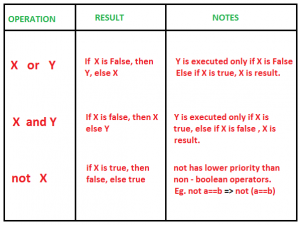Open in App
Not now

# Short Circuiting Techniques in Python

• Difficulty Level : Medium
• Last Updated : 15 May, 2022

By short-circuiting, we mean the stoppage of execution of boolean operation if the truth value of expression has been determined already. The evaluation of expression takes place from left to right. In python, short-circuiting is supported by various boolean operators and functions.

Short-Circuiting in Boolean Operators

The chart given below gives an insight into the short-circuiting case of boolean expressions. Boolean operators are ordered by ascending priority.or: When the Python interpreter scans or expression, it takes the first statement and checks to see if it is true. If the first statement is true, then Python returns that object’s value without checking the second statement. The program does not bother with the second statement. If the first value is false, only then Python check the second value, and then the result is based on the second half.
and: For an and expression, Python uses a short circuit technique to check if the first statement is false then the whole statement must be false, so it returns that value. Only if the first value is true, does it check the second statement and return the value.
An expression containing and or stops execution when the truth value of expression has been achieved. Evaluation takes place from left to right.

## Python3

 `# python code to demonstrate short circuiting ``# using and and or``  ` `# helper function``def` `check():``    ``return` `"geeks"``  ` `# using an expression to demonstrate``# prints "geeks", and gets executed ``# as both are required to check truth value``print` `(``1` `and` `check())``  ` `      ` `# using an expression to demonstrate``# prints 1``# as only if 1st value is true, or ``# doesnt require call check() fun``print` `(``1` `or` `check())``  ` `# using an expression to demonstrate``# prints "geeks"``# the value returns true when check ``# is encountered. 1 is not executed``print` `(``0` `or` `check() ``or` `1``)``  ` `# using an expression to demonstrate``# prints 1``# as last value is required to evaluate``# full expression because of "and"``print` `(``0` `or` `check() ``and` `1``)`

Output

```geeks
1
geeks
1
```
Short-Circuiting in all() and any()

Inbuilt functions all() and any() in python also support short-circuiting. The example below would give you clear insight into how it works.

## Python3

 `# python code to demonstrate short circuiting ``# using all() and any()``  ` `# helper function``def` `check(i):``    ``print` `(``"geeks"``)``    ``return` `i``  ` `# using all()``# stops execution when false occurs``# tells the compiler that even if one is ``# false, all cannot be true, hence stop ``# execution further.``# prints 3 "geeks" ``print` `(``all``(check(i) ``for` `i ``in` `[``1``, ``1``, ``0``, ``0``, ``3``]))``  ` `print``(``"\r"``)``  ` `# using any()``# stops execution when true occurs``# tells the compiler that even if one is ``# true, expression is true, hence stop ``# execution further.``# prints 4 "geeks" ``print` `(``any``(check(i) ``for` `i ``in` `[``0``, ``0``, ``0``, ``1``, ``3``]))`

Output

```geeks
geeks
geeks
False

geeks
geeks
geeks
geeks
True
```
Short-Circuiting in conditional operators

Conditional operators also follow short-circuiting as when expression result is obtained, further execution is not required.

## Python3

 `# python code to demonstrate short circuiting ``# using conditional operators``  ` `# helper function``def` `check(i):``    ``print` `(``"geeks"``)``    ``return` `i``  ` `# using conditional expressions``# since 10 is not greater than 11``# further execution is not taken place ``# to check for truth value.``print``( ``10` `> ``11` `> check(``3``) )``  ` `print` `(``"\r"``)``  ` `# using conditional expressions``# since 11 is greater than 10``# further execution is taken place ``# to check for truth value.``# return true as 11 > 3``print``( ``10` `< ``11` `> check(``3``) )``  ` `  ` `print` `(``"\r"``)``  ` `  ` `# using conditional expressions``# since 11 is greater than 10``# further execution is taken place ``# to check for truth value.``# return false as 11 < 12``print``( ``10` `< ``11` `> check(``12``) )`

Output

```False

geeks
True

geeks
False
```

Short-circuiting in a ladder of if, elif, elif, … else statements

When there is a sequence, aka a “ladder” of if and one or more elif statements, none of the conditions after the first one found to be True are evaluated.

## Python3

 `a ``=` `10``b ``=` `20``c ``=` `30` `def` `printreturn(l):``   ``print``(l)``   ``return` `l` `if` `a ``=``=` `11``:``    ``print``(``"a == 11"``)``elif` `b ``=``=` `20` `and` `c ``=``=` `30``: ``# This is True``    ``print``(``"b==20 and c==30"``)``elif` `b ``=``=` `a``+``a ``and` `0``<``len``(printreturn(``"This was evaluated"``)):``     ``# Though True, this is not even evaluated``    ``print``(``"b == a+a"``)`

Output

```b==20 and c==30
```

This article is contributed by Manjeet Singh. If you like GeeksforGeeks and would like to contribute, you can also write an article using write.geeksforgeeks.org or mail your article to review-team@geeksforgeeks.org. See your article appearing on the GeeksforGeeks main page and help other Geeks.